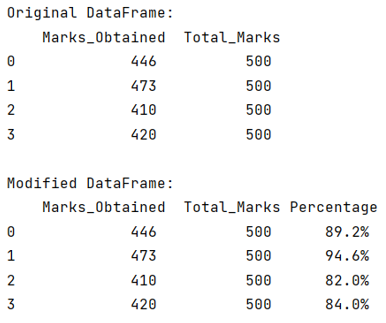# Make new column in Pandas DataFrame by adding values from other columns

Given a Pandas DataFrame, we have to make new column by adding values from other columns.
Submitted by Pranit Sharma, on July 23, 2022

Pandas is a special tool that allows us to perform complex manipulations of data effectively and efficiently. Inside pandas, we mostly deal with a dataset in the form of DataFrame. DataFrames are 2-dimensional data structures in pandas. DataFrames consist of rows, columns, and data.

Given a DataFrame, we need to create a new column that contains the value which is generated by performing some operation between the other column values.

To create a new column in pandas DataFrame which contains the value generated by performing some operation on the values of other columns, we will first create a DataFrame, the dataframe will contain 2 columns initially and we will perform a row-wise product of both columns and store all the values in a list. Finally, we will assign this list to a new column of the DataFrame.

To work with pandas, we need to import pandas package first, below is the syntax:

```import pandas as pd
```

Let us understand with the help of an example,

## Python code to make new column in Pandas DataFrame by adding values from other columns

```# Importing pandas package
import pandas as pd

# Creating a Dictionary
d = {
'Marks_Obtained':[446,473,410,420],
'Total_Marks': [500,500,500,500]
}

# Creating a DataFrame
df = pd.DataFrame(d)

# Display original DataFrame
print("Original DataFrame:\n",df,"\n")

# Creating a list of values
l = []
for i in range(len(df['Marks_Obtained'])):
a = (df['Marks_Obtained'][i]/df['Total_Marks'][i])*100
l.append(str(a)+'%')

# Creating a new column and assigning its values
# as the list defined above
df['Percentage'] = l

# Display modified DataFrame
print("Modified DataFrame:\n",df)
```

Output:Top MCQs